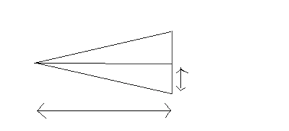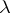## Double Slit Diffraction

### Young’s Double Slit Experiment (1801)

Click below to see a demonstration of Young’s Experiment.

Young’s Experiment

Vary the slit distance and notice what happens to the pattern on the screen.

Several things should be emphasized from this double slit setup:

• A maximum bright line is formed in the geometric center, caused by constructive interference of the light waves – called the principal image or central bright spot.
• Other bright lines are formed which have a smaller intensity than the center bright line. These are called the second, third, fourth, (and so on) images.
• Dark lines are formed between the bright lines where destructive interference has occurred.
• Decreasing the slit separation increases the width of the bright and dark bands.The light source would be located on the left side, as would the diffraction grating. The diffraction grating is a glass or plastic material that has series of very closely spaced slits. A diffraction grating constant is assigned to every grating. This is known as “d”.

The horizontal arrow represents how far the diffraction grating is placed with respect to the screen. This is known as “L”.

The vertical arrow represents the location of the first order image with respect to the principal image. This is known as “y”. (sometimes seen as “x” in some equations)

The screen is located on the right side of the diagram, on which “y” is measured.
The angle, theta, must also be known (or calculated)…this is the smaller angle on the left side for one of the triangles.

The diagram can be simplified even more than above by just drawing one right triangle (instead of the two shown).

## Equations Used for Double Slit Interference= (d sin)/n= wavelength of light, in m

d = diffraction grating constant, in m= angle between line drawn to first order image and straight line to principal image

n = image order, 1,2,3,etc (maximum brightness)

[Use (n + 1/2) for minimums, where n = 0,1,2, etc.]

Formulas for positions of dark and bright bands:

Ybright = (L n)/d

Ydark = [L(n + 1/2)]/d

## Diffraction Problems Quiz

Open and save the document from the sidebar handout titled Diffraction Problems. After you have completed the diffraction problems and feel confident with the material, you will be prepared for a quiz over this material.

## Single Slit Diffraction

Click on the following Single Slit Diffraction Exercise. Notice what happens to the distance between the images when the wavelength of the light is changed.

Single Slit Diffraction Exercise

Some important points about the single slit pattern formed:

• The central bright band is twice the width of any other order image.
• The light intensities decrease as the pattern moves away from the central bright band.

A right triangle can be drawn as in double slit diffraction problems. The equation used for single slit diffraction is

sin= m/a= angle between straight line path to screen and straight line path to the first order image.

m = band order of the image 1,2,3, etc.= wavelength of light, m

a = slit width, in m

(source)